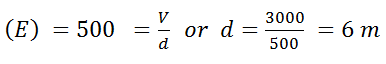# From a point charge, there is a fixed point A. At A, there is an electric field of 500 V/m and potential difference of 3000 V. Distance between point charge and A will be         (a)    6 m         (b)    12 m         (c)    16 m         (d)    24 m

8 years ago

(a)

Given: Electric field (E) = 500 V/m and potential difference

(V) = 3000 V.

We know that electric field[where d = Distance between point charge and A]